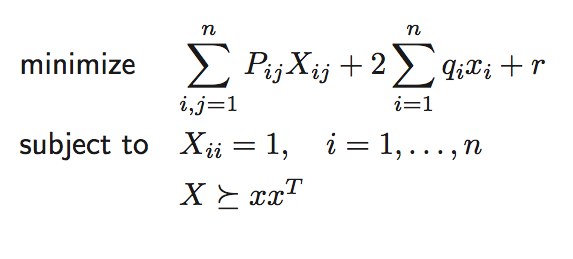# Urgent help with a problem with CVX

I would like to solve the following optimization problem with CVXAfter initializing P, q, r, n, I tried the following in matlab.

cvx_begin
variables W(n,n) x(n);
minimize(sum(sum(P.W)) + 2sum(q.x) + r);
subject to;
diag(W) == 1;
W == semidefinite(n)+x
x’;
cvx_end

I see the following error. It is clear the error is due to the term x*x’. However, I don’t know how to fix it. Any help will be appreciated. Thanks!

In cvxprob (line 28)
In cvx_begin (line 41)
Error using * (line 126)
Disciplined convex programming error:
Only scalar quadratic forms can be specified in CVX

You need to use a Schur complement. See https://web.stanford.edu/~boyd/cvxbook/bv_cvxbook.pdf .

[W x;x’ 1] == semidefinite(n+1)